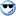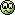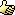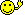CodeGuru Home VC++ / MFC / C++ .NET / C# Visual Basic VB Forums Developer.com

# Thread: [RESOLVED] An error in my code - binary digit matrix implementation by using 2D vector

1.MemberJoin Date
Mar 2018
Posts
40

## [RESOLVED] An error in my code - binary digit matrix implementation by using 2D vector

Hello

[/HTML]
Last edited by Kmilano; April 23rd, 2018 at 09:51 AM.Reply With Quote

2. ## Re: An error in my code - binary digit matrix implementation by using 2D vectorOriginally Posted by Kmilano...
when I use vectors which I commented in the following code without void function everything is okay and if I want to merge them into one vector like following with void function I will have an error in this line:

HTML Code:
`for (const auto& row : generate_mtx(nbits))`
1. Please, post the exact error text.
2. Please, use CODE tags, not the HTML ones!Reply With Quote

3.MemberJoin Date
Mar 2018
Posts
40

## Re: An error in my code - binary digit matrix implementation by using 2D vector

the errors:
1
main.cpp||In function ‘int main()’:|
2
main.cpp|40|error: invalid initialization of reference of type ‘std::vector<std::vector<int> >&’ from exp<b></b>ression of type ‘size_t {aka long unsigned int}’|
3
main.cpp|12|note: in passing argument 1 of ‘void generate_mtx(std::vector<std::vector<int> >&, std::size_t)’|
4
main.cpp|42|error: unable to deduce ‘auto&&’ from ‘row’|
5
||=== Build failed: 2 error(s), 0 warning(s) (0 minute(s), 0 second(s)) ===|
Last edited by Kmilano; April 23rd, 2018 at 09:52 AM.Reply With Quote

4. ## Re: An error in my code - binary digit matrix implementation by using 2D vectorOriginally Posted by Kmilanothe errors:
1
main.cpp||In function ‘int main()’:|
2
main.cpp|40|error: invalid initialization of reference of type ‘std::vector<std::vector<int> >&’ from exp<b></b>ression of type ‘size_t {aka long unsigned int}’|
...
Code:
```...
for (const auto& row : generate_mtx(nbits))
{
for (int v : row) std::cout << v << ' ';
std::cout << '\n';
}
...```
Your code refers to the function generate_mtx(nbits), however the only function with this name is declared as void, so it cannot return anything...
Sure, it is not what you expected!Reply With Quote

5.MemberJoin Date
Mar 2018
Posts
40

## Re: An error in my code - binary digit matrix implementation by using 2D vector

in fact,
Last edited by Kmilano; April 23rd, 2018 at 09:51 AM.Reply With Quote

6. ## Re: An error in my code - binary digit matrix implementation by using 2D vectorOriginally Posted by Kmilanoin fact, first my code was like the following code and it works correctly, but because I must use void function to match base class with a derived class, so I changes that line to void function,
now, what's a solution to solve this error?
You could implement the overloaded method in your derived class that would either return your created vector or you would pass this vector as a parameter by reference into your method.
Variant 1 (pseudo code):
Code:
```typedef std::vector< std::vector<int> > myMatrix;

myMatrix generate_mtx(std::size_t nbits)
{
nbits %= std::numeric_limits<unsigned long long>::digits;

myMatrix result(nbits);

// note: col with all zeroes is skipped (start with number = 0 to include it)
for (unsigned long long number = 1; number < ubound(nbits); ++number)
{
auto n = number;
for (auto& vec : result)
{
vec.push_back(n % 2);
n /= 2;
}
}
std::reverse(std::begin(result), std::end(result));

return result;
}```
Variant 2 (pseudo code):
Code:
```typedef std::vector< std::vector<int> > myMatrix;

myMatrix generatedMatrix;
void generate_mtx(std::size_t nbits, myMatrix& generatedMatrix)
{
nbits %= std::numeric_limits<unsigned long long>::digits;

// note: col with all zeroes is skipped (start with number = 0 to include it)
for (unsigned long long number = 1; number < ubound(nbits); ++number)
{
auto n = number;
for (auto& vec : generatedMatrix)
{
vec.push_back(n % 2);
n /= 2;
}
}
std::reverse(std::begin(generatedMatrix), std::end(generatedMatrix));
}```Reply With Quote

7. ## Re: An error in my code - binary digit matrix implementation by using 2D vector

Code:
`for (const auto& row : generate_mtx(nbits))`
When using a range-based for loop, the range expression (the return type of generate_mtx(nbits) in this case) needs to be of a type that supports the begin() and end() iterator functions so that the range can be obtained. vector<> does but void doesn't. If generate_mtx() is required to return a void, then generate_mtx() needs to have a reference parameter as Victor uses in Variant 2 in post #6. In this case the range_for would be something like (not tried)

Code:
```typedef std::vector< std::vector<int> > myMatrix;

myMatrix generatedMatrix;
generate_mtx(nbits, generatedMatrix);

for (const auto& row : generatedMatrix)
{
for (int v : row)
std::cout << v << ' ';

std::cout << '\n';
}```Reply With Quote

8.MemberJoin Date
Mar 2018
Posts
40

## Re: An error in my code - binary digit matrix implementation by using 2D vector

thank you for your response, but I cannot use matrix and I must use the code which exists in my first post, In my last reply I posted my original correct code, but I cannot use it,
if its possible please give me an alternative way for correcting the first code:
Last edited by Kmilano; April 23rd, 2018 at 09:52 AM.Reply With Quote

9. ## Re: An error in my code - binary digit matrix implementation by using 2D vector

[QUOTE=Kmilano;2221795]thank you for your response, but I cannot use matrix
What "matrix" do you mean?if it is the one I referred in my post asOriginally Posted by Victor
Code:
`typedef std::vector< std::vector<int> > myMatrix;`
then sorry! It is just a typedef! I used it to make code simpler (to write and to understand!)Originally Posted by Kmilanoif its possible please give me an alternative way for correcting the first code:

Code:
```#include "stdafx.h"
#include <iostream>
#include <limits>
#include <vector>
#include <algorithm>
using namespace std;

constexpr unsigned long long ubound(std::size_t nbits)
{
if (nbits < 2) return 2;
else return ubound(nbits - 1) * 2;
}

//std::vector< std::vector<int> > generate_mtx(std::size_t nbits)
void generate_mtx(std::vector< std::vector<int> >& result, std::size_t nbits)
{
nbits %= std::numeric_limits<unsigned long long>::digits;

//std::vector< std::vector<int> > result(nbits);

// note: col with all zeroes is skipped (start with number = 0 to include it)
for (unsigned long long number = 1; number < ubound(nbits); ++number)
{
auto n = number;
for (auto& vec : result)
{
vec.push_back(n % 2);
n /= 2;
}
}

// to get the rows in the same order as illustrated in the example
std::reverse(std::begin(result), std::end(result));

//return result;
}

int main()
{

if (size_t nbits = 4)
{
std::cout << "check matrix (H) is: " << std::endl;

for (const auto& row : generate_mtx(nbits))
{
for (int v : row) std::cout << v << ' ';
std::cout << '\n';
}

}
int pause;
std::cin >> pause;
//return 0;
}```
I did it in my previous post.Alternatively you could define your "matrix" (std::vector< std::vector<int> >) as a global object but in C++ it is not recommended.Reply With Quote

10. ## Re: An error in my code - binary digit matrix implementation by using 2D vector

I think this is what you are after. It produces the required output.

Code:
```#include <iostream>
#include <limits>
#include <vector>
#include <algorithm>
using namespace std;

constexpr unsigned long long ubound(std::size_t nbits)
{
if (nbits < 2) return 2;
else return ubound(nbits - 1) * 2;
}

void generate_mtx(std::vector< std::vector<int> >& result, std::size_t nbits)
{
nbits %= std::numeric_limits<unsigned long long>::digits;
result.resize(nbits);

// note: col with all zeroes is skipped (start with number = 0 to include it)
for (unsigned long long number = 1; number < ubound(nbits); ++number)
{
auto n = number;
for (auto& vec : result)
{
vec.push_back(n % 2);
n /= 2;
}
}

// to get the rows in the same order as illustrated in the example
std::reverse(std::begin(result), std::end(result));
}

int main()
{
if (size_t nbits = 4)
{
std::vector< std::vector<int> > results;

std::cout << "check matrix (H) is: " << std::endl;
generate_mtx(results, nbits);

for (const auto& row : results)
{
for (int v : row) std::cout << v << ' ';
std::cout << '\n';
}
}

std::cin.get();
}```
Code:
```check matrix (H) is:
0 0 0 0 0 0 0 1 1 1 1 1 1 1 1
0 0 0 1 1 1 1 0 0 0 0 1 1 1 1
0 1 1 0 0 1 1 0 0 1 1 0 0 1 1
1 0 1 0 1 0 1 0 1 0 1 0 1 0 1```
Last edited by 2kaud; March 25th, 2018 at 12:25 PM.Reply With Quote

11.MemberJoin Date
Mar 2018
Posts
40

## Re: An error in my code - binary digit matrix implementation by using 2D vector

yes thank you so much, its my desired codeReply With Quote

#### Tags for this Thread

c++####Posting Permissions

• You may not post new threads
• You may not post replies
• You may not post attachments
• You may not edit your posts
•Click Here to Expand Forum to Full Width